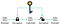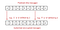Secret Key Algorithms in Cryptography

Cryptography is the study and practice of hiding data. It provides the services : Confidentiality, Integrity, Authentication and Non-repudiation.

Cryptography is mainly focused on securing data at transit but it can be used for securing data at rest too. It is heavily used in technical controls.

Cryptography ensures that the message sent by the authorized sender is only received by the authorized recipient. Any other party should not be able to read the message.

A plain text, which is the meaningful and useful message is encrypted using an encryption algorithm to create a cipher text. The cipher text thus created, is a meaningless unreadable message. The encryption algorithm uses a key for encrypting. The same key is needed to decrypt and obtain the plain text again.

Most modern encryption algorithms use a key. It is preferable to use a key because the algorithm can even be published while keeping the key a secret.

Some algorithms do not use a key, but such algorithms can be easily cracked using reverse engineering mechanisms.

Secret Key Algorithms

A secret key algorithm or symmetric encryption algorithm uses a copy or instance of the same key to encrypt and decrypt. Generally it uses keys of 128–256 bit long size. Symmetric encryption is fast because data can be encrypted on the go and because the key size is small. Therefore, for bulk data encryption, secret key algorithms are used.Secret Key Algorithms use the same key for encryption and decryption

One of the simplest examples of a secret key algorithm is the Caeser’s Cipher. Each letter of the message is shifted using a key. For example, if key=2, A would be replaced by B, C would be replaced by D etc. This encryption method is highly vulnerable as the secret key should be shared and also we need to ensure that the shared key is secured.Look how the message ‘attack at dawn’ is encrypted using the key 2

The receiver of the message should know the key in order to decrypt the ciphertext. For this purpose, the key should also be shared along with the encrypted message.

Some examples of Secrete Key Algorithms : DES (Data Encryption Standard), 3DES (Tripe Data Encryption Standard), AES (Advanced Encryption Standard), IDEA (International Data Encryption Algorithm) and Blowfish.

Now let’s look at some of these algorithms.

Data Encryption Standard

DES has significantly lost popularity as it has been found very vulnerable against powerful attacks. DES uses the same 56-bit key to encrypt data blocks of 64-bits with the same algorithm.

DES has several modes of operations.

1 ECB — Electronic Code Book

In ECB, parallel encryption and decryption is possible. It is a simple mode and is suitable for encrypting small amounts of data. Each block of data is encrypted using the same key.

One of the drawbacks this mode has is, similar blocks of plain text will give the same cipher text blocks. When a large amount of data is available, these patterns will retain in the cipher text blocks too. This makes it easier for cryptanalysis.

2 CBC — Cipher Block Chaining

This mode is complex but secure than ECB. The mode uses an Initial Vector — IV as the first key and the first block of data is “XOR-ed” (IV can be any bit pattern)

The cipher text of the first block is then used to XOR the second block of data. The cipher text of the second block is used to XOR the third block of data and it goes on in this way.

Simply, Each block is “XOR-ed” with the cipher text of the previous block except for the first block where an IV is used.

The advantage of CBC over ECB is, even if similar blocks of data are available, the cipher text of them would be different and it will be difficult for cryptanalysis.

Some drawback of CBC are because of complexity more processing speed is needed and if an error occurs in encrypting one block, it will be propagated to others blocks as well.

Triple Data Encryption Standard

Triple Data Encryption Standard or 3-DS is simply three times DES and is enhancement of DES.

The algorithm uses 2 or more different keys to encrypt/decrypt 3 times.

In the above figure, the plain text is encrypted using K1, the output is encypted again with another key K2 followed by the another encryption with key K3.

There are several modes in 3-DES

2 EDE — 3DES Using 2 keys and follow encrypt, decrypt, encrypt order

3 EDE — 3DES Using 3 keys and follow encrypt, decrypt, encypt order

2 EEE — 3DES Using 2 keys and follow encrypt, encrypt, encrypt order

3 EEE — 3DES Using 3 keys and follow encrypt, encrypt, encrypt order

AES or Advanced Encryption Standard is the standard used currently. It uses 3 keys sizes : 128-bit, 192-bit and 256-bit of which 128-bit is the most commonly used. AES was introduced as the key size in DES was too small.

In AES, each round comprises of 4 sub-processes.

1. Byte-substitution (SubBytes)
2. Shiftrows
3. MixColumns